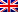# External quality criteria

Contenus

## External quality criteria

External quality criteria (metric based on mutual information, precision recall metric, RAND index) can be useful in examining whether the cluster structure matches a predefined classification of instances. The external quality criteria are explained below.

## Measure based on mutual information

The mutual information criterion can be used as an external measure for clustering. The measure for m instances grouped together using C = {C_1 ,. . . , C_g} and referring to the target attribute y whose domain is dom (y) = {c_1 ,. . . , c_k} is defined as follows:

where m_l, h indicates the number of instances that are in cluster C_l and also in class c_h. m., h indicates the total number of instances in class c_h. Likewise, m_l ,. indicates the number of instances of the C_l cluster.

MI is combined with entropy in the NMI:

MI is combined with entropy in AMI:

## Precision recall measurement

The precision recall metric from information research can be used as an external metric to assess clusters. The cluster is seen as the result of a query for a specific class. Precision is the fraction of correctly fetched instances, while recall is the fraction of successfully fetched instances of all matching instances. A combined F-measure can be useful for evaluating a clustering structure.

## Rand index

The Rand index is a simple criterion used to compare an induced aggregation structure (C1) with a given aggregation structure (C2). Let a be the number of pairs of instances assigned to the same cluster in C1 and in the same cluster in C2; let b be the number of pairs of instances which are in the same cluster C1, but not in the same cluster C2; let c be the number of pairs of instances which are in the same cluster C2, but not in the same cluster C1; and d the number of pairs of instances allocated to different clusters than C1 and C2.

The quantities a and d can be interpreted as agreements, and b and c as disagreements. The Rand index is defined as:

The Rand index is between 0 and 1. When the two partitions match perfectly, the Rand index is 1.

One problem with the Rand index is that its expected value of two random groupings does not take a constant value (such as zero). Hubert and Arabia in 1985 suggest an adjusted Rand index which overcomes this drawback.FRFRENRUES